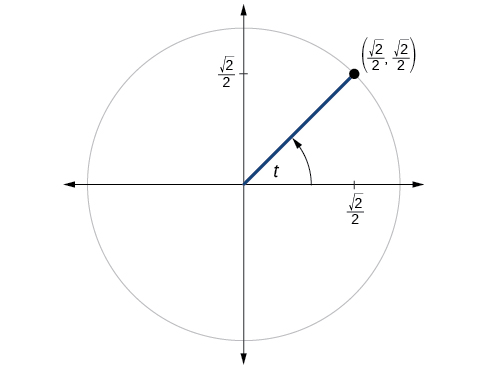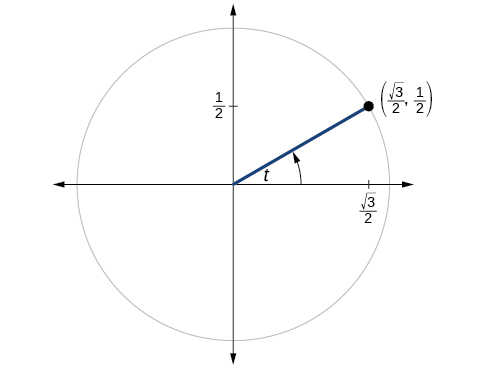# 7.4 The other trigonometric functions  (Page 7/14)

 Page 7 / 14

If $\mathrm{tan}\text{\hspace{0.17em}}t=\frac{12}{5},$ and $\text{\hspace{0.17em}}0\le t<\frac{\pi }{2},$ find $\text{\hspace{0.17em}}\mathrm{sin}\text{\hspace{0.17em}}t,\mathrm{cos}\text{\hspace{0.17em}}t,\mathrm{sec}\text{\hspace{0.17em}}t,\mathrm{csc}\text{\hspace{0.17em}}t,\text{and}\text{\hspace{0.17em}}\mathrm{cot}\text{\hspace{0.17em}}t.$

If $\text{\hspace{0.17em}}\mathrm{sin}\text{\hspace{0.17em}}t=\frac{\sqrt{3}}{2}\text{\hspace{0.17em}}$ and $\text{\hspace{0.17em}}\mathrm{cos}\text{\hspace{0.17em}}t=\frac{1}{2},$ find $\text{\hspace{0.17em}}\mathrm{sec}\text{\hspace{0.17em}}t,\mathrm{csc}\text{\hspace{0.17em}}t,\mathrm{tan}\text{\hspace{0.17em}}t,$ and $\text{\hspace{0.17em}}\mathrm{cot}\text{\hspace{0.17em}}t.$

$\mathrm{sec}t=2,$ $\mathrm{cot}t=\frac{\sqrt{3}}{3}$

If $\text{\hspace{0.17em}}\mathrm{sin}\text{\hspace{0.17em}}40°\approx 0.643\text{\hspace{0.17em}}$ and $\text{\hspace{0.17em}}\mathrm{cos}\text{\hspace{0.17em}}40°\approx 0.766,$ find $\text{\hspace{0.17em}}\text{sec}\text{\hspace{0.17em}}40°,\text{csc}\text{\hspace{0.17em}}40°,\text{tan}\text{\hspace{0.17em}}40°,$ and $\text{\hspace{0.17em}}\text{cot}\text{\hspace{0.17em}}40°.$

If $\text{\hspace{0.17em}}\text{sin}\text{\hspace{0.17em}}t=\frac{\sqrt{2}}{2},$ what is the $\text{\hspace{0.17em}}\text{sin}\left(-t\right)?$

$-\frac{\sqrt{2}}{2}$

If $\text{\hspace{0.17em}}\text{cos}\text{\hspace{0.17em}}t=\frac{1}{2},$ what is the $\text{\hspace{0.17em}}\text{cos}\left(-t\right)?$

If $\text{\hspace{0.17em}}\text{sec}\text{\hspace{0.17em}}t=3.1,$ what is the $\text{\hspace{0.17em}}\text{sec}\left(-t\right)?$

3.1

If $\text{\hspace{0.17em}}\text{csc}\text{\hspace{0.17em}}t=0.34,$ what is the $\text{\hspace{0.17em}}\text{csc}\left(-t\right)?$

If $\text{\hspace{0.17em}}\text{tan}\text{\hspace{0.17em}}t=-1.4,$ what is the $\text{\hspace{0.17em}}\text{tan}\left(-t\right)?$

1.4

If $\text{\hspace{0.17em}}\text{cot}\text{\hspace{0.17em}}t=9.23,$ what is the $\text{\hspace{0.17em}}\text{cot}\left(-t\right)?$

## Graphical

For the following exercises, use the angle in the unit circle to find the value of the each of the six trigonometric functions.$\mathrm{sin}t=\frac{\sqrt{2}}{2},\mathrm{cos}t=\frac{\sqrt{2}}{2},\mathrm{tan}t=1,\mathrm{cot}t=1,\mathrm{sec}t=\sqrt{2},\mathrm{csc}t=\sqrt{2}$$\mathrm{sin}t=-\frac{\sqrt{3}}{2},\mathrm{cos}t=-\frac{1}{2},\mathrm{tan}t=\sqrt{3},\mathrm{cot}t=\frac{\sqrt{3}}{3},\mathrm{sec}t=-2,\mathrm{csc}t=-\frac{2\sqrt{3}}{3}$

## Technology

For the following exercises, use a graphing calculator to evaluate to three decimal places.

$\mathrm{csc}\text{\hspace{0.17em}}\frac{5\pi }{9}$

$\mathrm{cot}\text{\hspace{0.17em}}\frac{4\pi }{7}$

–0.228

$\mathrm{sec}\text{\hspace{0.17em}}\frac{\pi }{10}$

$\mathrm{tan}\text{\hspace{0.17em}}\frac{5\pi }{8}$

–2.414

$\mathrm{sec}\text{\hspace{0.17em}}\frac{3\pi }{4}$

$\mathrm{csc}\text{\hspace{0.17em}}\frac{\pi }{4}$

1.414

$\text{tan}\text{\hspace{0.17em}}98°$

$\mathrm{cot}\text{\hspace{0.17em}}33°$

1.540

$\mathrm{cot}\text{\hspace{0.17em}}140°$

$\mathrm{sec}\text{\hspace{0.17em}}310°$

1.556

## Extensions

For the following exercises, use identities to evaluate the expression.

If $\text{\hspace{0.17em}}\mathrm{tan}\left(t\right)\approx 2.7,$ and $\text{\hspace{0.17em}}\mathrm{sin}\left(t\right)\approx 0.94,$ find $\text{\hspace{0.17em}}\mathrm{cos}\left(t\right).$

If $\text{\hspace{0.17em}}\mathrm{tan}\left(t\right)\approx 1.3,$ and $\text{\hspace{0.17em}}\mathrm{cos}\left(t\right)\approx 0.61,$ find $\text{\hspace{0.17em}}\mathrm{sin}\left(t\right).$

$\mathrm{sin}\left(t\right)\approx 0.79$

If $\text{\hspace{0.17em}}\mathrm{csc}\left(t\right)\approx 3.2,$ and $\text{\hspace{0.17em}}\mathrm{cos}\left(t\right)\approx 0.95,$ find $\text{\hspace{0.17em}}\mathrm{tan}\left(t\right).$

If $\text{\hspace{0.17em}}\mathrm{cot}\left(t\right)\approx 0.58,$ and $\text{\hspace{0.17em}}\mathrm{cos}\left(t\right)\approx 0.5,$ find $\text{\hspace{0.17em}}\mathrm{csc}\left(t\right).$

$\mathrm{csc}t\approx 1.16$

Determine whether the function $\text{\hspace{0.17em}}f\left(x\right)=2\mathrm{sin}x\text{\hspace{0.17em}}\mathrm{cos}\text{\hspace{0.17em}}x\text{\hspace{0.17em}}$ is even, odd, or neither.

Determine whether the function $\text{\hspace{0.17em}}f\left(x\right)=3{\mathrm{sin}}^{2}x\text{\hspace{0.17em}}\mathrm{cos}\text{\hspace{0.17em}}x+\mathrm{sec}\text{\hspace{0.17em}}x\text{\hspace{0.17em}}$ is even, odd, or neither.

even

Determine whether the function $\text{\hspace{0.17em}}f\left(x\right)=\mathrm{sin}\text{\hspace{0.17em}}x-2{\mathrm{cos}}^{2}x\text{\hspace{0.17em}}$ is even, odd, or neither.

Determine whether the function $\text{\hspace{0.17em}}f\left(x\right)={\mathrm{csc}}^{2}x+\mathrm{sec}\text{\hspace{0.17em}}x\text{\hspace{0.17em}}$ is even, odd, or neither.

even

For the following exercises, use identities to simplify the expression.

$\mathrm{csc}\text{\hspace{0.17em}}t\text{\hspace{0.17em}}\mathrm{tan}\text{\hspace{0.17em}}t$

$\frac{\mathrm{sec}\text{\hspace{0.17em}}t}{\mathrm{csc}\text{\hspace{0.17em}}t}$

$\frac{\mathrm{sin}\text{\hspace{0.17em}}t}{\mathrm{cos}\text{\hspace{0.17em}}t}=\mathrm{tan}\text{\hspace{0.17em}}t$

## Real-world applications

The amount of sunlight in a certain city can be modeled by the function $\text{\hspace{0.17em}}h=15\mathrm{cos}\left(\frac{1}{600}d\right),$ where $\text{\hspace{0.17em}}h\text{\hspace{0.17em}}$ represents the hours of sunlight, and $\text{\hspace{0.17em}}d\text{\hspace{0.17em}}$ is the day of the year. Use the equation to find how many hours of sunlight there are on February 10, the 42 nd day of the year. State the period of the function.

The amount of sunlight in a certain city can be modeled by the function $\text{\hspace{0.17em}}h=16\mathrm{cos}\left(\frac{1}{500}d\right),$ where $\text{\hspace{0.17em}}h\text{\hspace{0.17em}}$ represents the hours of sunlight, and $\text{\hspace{0.17em}}d\text{\hspace{0.17em}}$ is the day of the year. Use the equation to find how many hours of sunlight there are on September 24, the 267th day of the year. State the period of the function.

13.77 hours, period: $\text{\hspace{0.17em}}1000\pi$

The equation $\text{\hspace{0.17em}}P=20\mathrm{sin}\left(2\pi t\right)+100\text{\hspace{0.17em}}$ models the blood pressure, $\text{\hspace{0.17em}}P,$ where $\text{\hspace{0.17em}}t\text{\hspace{0.17em}}$ represents time in seconds. (a) Find the blood pressure after 15 seconds. (b) What are the maximum and minimum blood pressures?

The height of a piston, $\text{\hspace{0.17em}}h,$ in inches, can be modeled by the equation $\text{\hspace{0.17em}}y=2\mathrm{cos}\text{\hspace{0.17em}}x+6,$ where $\text{\hspace{0.17em}}x\text{\hspace{0.17em}}$ represents the crank angle. Find the height of the piston when the crank angle is $\text{\hspace{0.17em}}55°.$

7.73 inches

The height of a piston, $\text{\hspace{0.17em}}h,$ in inches, can be modeled by the equation $\text{\hspace{0.17em}}y=2\mathrm{cos}\text{\hspace{0.17em}}x+5,$ where $\text{\hspace{0.17em}}x\text{\hspace{0.17em}}$ represents the crank angle. Find the height of the piston when the crank angle is $\text{\hspace{0.17em}}55°.$

## Angles

For the following exercises, convert the angle measures to degrees.

$45°$

$-\frac{5\pi }{3}$

For the following exercises, convert the angle measures to radians.

$-210°$

$-\frac{7\pi }{6}$

$180°$

Find the length of an arc in a circle of radius 7 meters subtended by the central angle of $\text{\hspace{0.17em}}85°.$

10.385 meters

the third and the seventh terms of a G.P are 81 and 16, find the first and fifth terms.
if a=3, b =4 and c=5 find the six trigonometric value sin
pls how do I factorize x⁴+x³-7x²-x+6=0
in a function the input value is called
how do I test for values on the number line
if a=4 b=4 then a+b=
a+b+2ab
Kin
commulative principle
a+b= 4+4=8
Mimi
If a=4 and b=4 then we add the value of a and b i.e a+b=4+4=8.
Tariq
what are examples of natural number
an equation for the line that goes through the point (-1,12) and has a slope of 2,3
3y=-9x+25
Ishaq
show that the set of natural numberdoes not from agroup with addition or multiplication butit forms aseni group with respect toaaddition as well as multiplication
x^20+x^15+x^10+x^5/x^2+1
evaluate each algebraic expression. 2x+×_2 if ×=5
if the ratio of the root of ax+bx+c =0, show that (m+1)^2 ac =b^2m
By the definition, is such that 0!=1.why?
(1+cosA+IsinA)(1+cosB+isinB)/(cos@+isin@)(cos$+isin$)
hatdog
Mark
jaks
Ryan
how we can draw three triangles of distinctly different shapes. All the angles will be cutt off each triangle and placed side by side with vertices touching

#### Get Jobilize Job Search Mobile App in your pocket Now!ByByBy OpenStaxBy OpenStaxByBy Janet ForresterBy Steve GibbsByBy Michael SagBy OpenStaxBy OpenStaxBy OpenStax